Function Repository Resource:

# PolygonNormalVector

Compute the normal vector to a 3D polygon

Contributed by: Jan Mangaldan
 ResourceFunction["PolygonNormalVector"][poly] gives the normal vector to the 3D polygon poly.

## Details and Options

poly can be a Polygon or a Triangle object.
The vector returned by ResourceFunction["PolygonNormalVector"] is always normalized to have unit length.
ResourceFunction["PolygonNormalVector"] accepts a Method option.
Method"Newell" uses Newell's method to compute the normal vector.
Method"SVD" uses singular value decomposition to compute the normal vector.

## Examples

### Basic Examples (2)

Compute the normal vector to a triangle:

 In:=Out=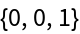Visualize the polygon and its normal:

 In:=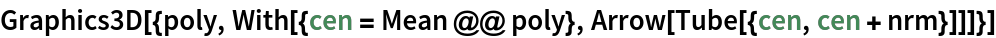Out=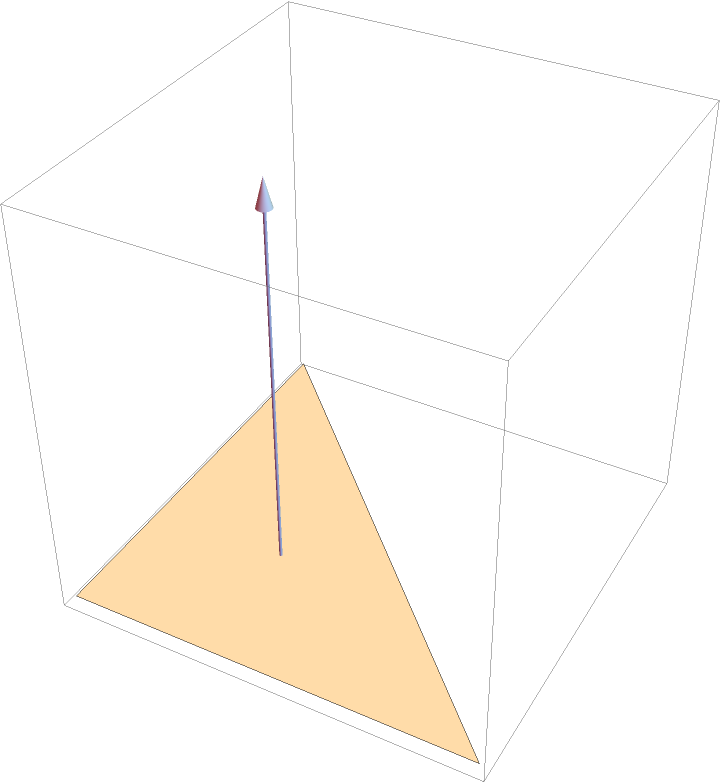### Scope (2)

PolygonNormalVector works on Triangle objects:

 In:=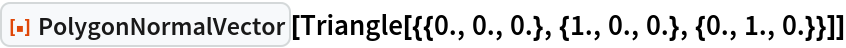Out=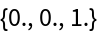PolygonNormalVector works on any 3D polygon:

 In:=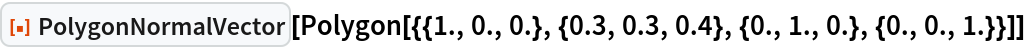Out=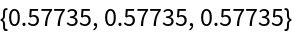### Options (1)

#### Method (1)

Compare the different methods of computing a normal vector:

 In:=In:=Out=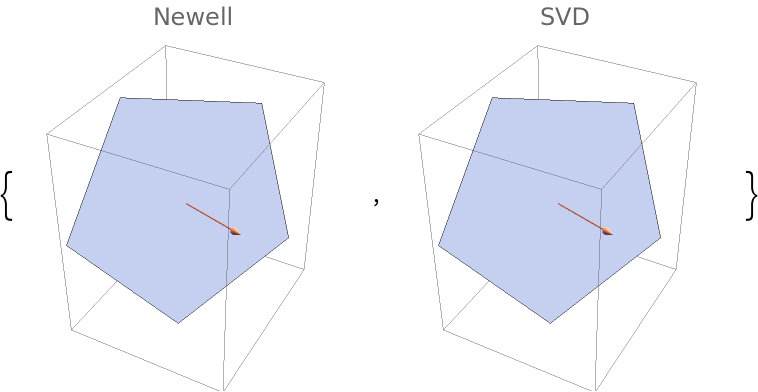### Applications (1)

Visualize the normal vectors for a model of Beethoven:

 In:=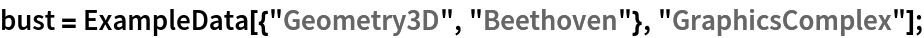In:=Out=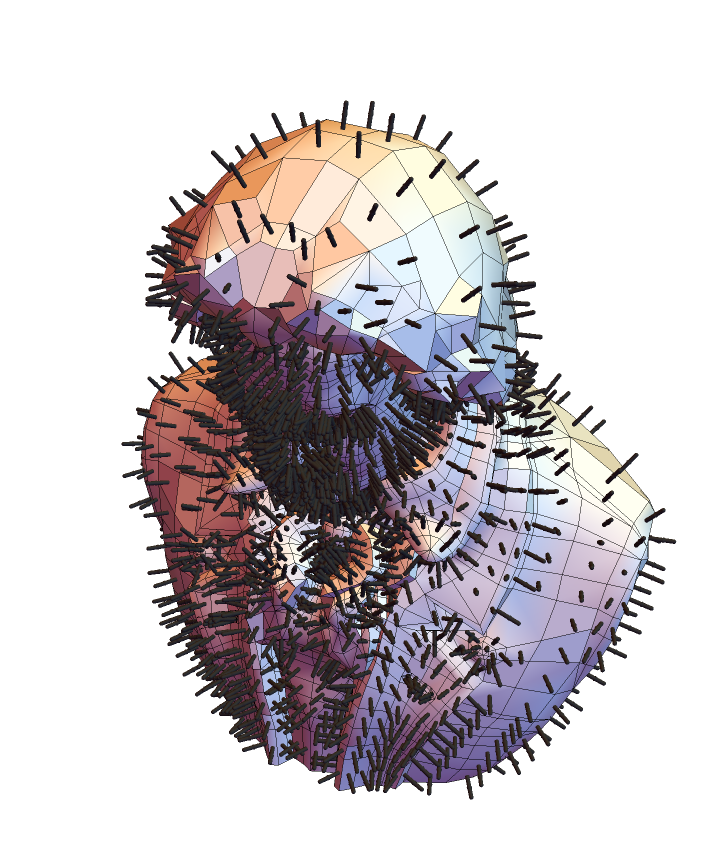## Version History

• 1.0.0 – 04 March 2021